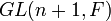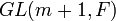# Difference between revisions of "Algebra group is isomorphic to algebra subgroup of unitriangular matrix group of degree one more than logarithm of order to base of field size"

## Statement

### Version for algebra group over prime field

Suppose$p$ is a prime number, and$G$ is a finite p-group, i.e., a group of order a power of$p$. Suppose$q$ is a power of$p$. Then, if$G$ is an algebra group over$\mathbb{F}_q$, the following must be true:

1. The order of$G$ is a power of$q$.
2.$G$ must be isomorphic to a subgroup of the unitriangular matrix group$UT(m + 1,q)$ where$m = \log_q(|G|)$, and the image of this isomorphism must have the further property that subtracting 1 from all its elements gives a subring of the nilpotent associative ring$NT(m + 1,q)$.

### Version for algebra group over prime field

Suppose$p$ is a prime number, and$G$ is a finite p-group, i.e., a group of order$p^n$ for some nonnegative integer$n$. Then, if$G$ is an algebra group over$\mathbb{F}_p$,$G$ must be isomorphic to a subgroup of the unitriangular matrix group$UT(n + 1,p)$. Further, the image of this isomorphism must have the further property that subtracting 1 from all its elements gives a subring of the nilpotent associative ring$NT(m + 1,q)$.

Note that if$G$ is an algebra group over any field extension of$\mathbb{F}_p$, it is also an algebra group over$\mathbb{F}_p$, so if this condition is violated,$G$ cannot be an algebra group over any$\mathbb{F}_q$ for$q$ a power of$p$.

## Facts used

1. Sylow's theorem, specifically Sylow implies order-dominating which says that any$p$-subgroup of a finite group is contained in a$p$-Sylow subgroup and hence has a conjugate contained in a predetermined$p$-Sylow subgroup.

## Proof

### Proof for finite field

Given:$G$ is a finite$p$-group that is an algebra group over$\mathbb{F}_q$, where$q$ is a power of$p$.

To prove: The order of$G$ is a power of$q$ and$G$ is isomorphic to a subgroup of$UT(m + 1,q)$ where$m = \log_q(|G|)$.

Step no. Assertion/construction Facts used Given data used Previous steps used Explanation
1 Let$N$ be a nilpotent associative algebra over$\mathbb{F}_q$ for which$G$ is the corresponding algebra group.$G$ is an algebra group.
2 The order of$G$ equals the order of$N$, and$m = \log_q(|G|)$ is an integer that equals the dimension of$N$ as a vector space over$\mathbb{F}_q$. Step (1)
3$N + F$ (the unitization of$N$) has dimension$m + 1$, and it acts faithfully on itself by left multiplication. Steps (1), (2)
4$N + F$ is isomorphic to a subring of the matrix ring$M(n + 1,F)$. Under this identification,$G$, as a multiplicative subgroup, is isomorphic to a multiplicative subgroup of$GL(n+1,F)$ and$N$ is a nilpotent subring of$M(n+1,F)$. Step (3)
5 The image of$G$ under the isomorphism of Step (4) is conjugate to a subgroup of$UT(m + 1,F)$, which is the$p$-Sylow subgroup of$GL(m+1,F)$. Moreover, subtracting 1 from all the elements of this subgroup gives a nilpotent associative subring of$NT(m+1,F)$ (the strictly upper triangular matrices). Fact (1)$G$ is a$p$-group Step (4) By Step (4) and Fact (1), we know that the image of$G$ in$GL(m + 1,F)$ is conjugate to a subgroup of$UT(m+1,F)$, the upper triangular matrices. Under this conjugation operation,$N$ goes to a new ring. Since subtracting 1 commutes with conjugation, this conjugate of$N$ is the same as the ring obtained by subtracting 1 from the conjugate of$G$. Hence, it is a subring of$UT(m+1,F)$.
6 The image of$G$ under the isomorphism of Step (4) is conjugate to a subgroup of$UT(m + 1,F)$ with the property that subtracting 1 from all its element gives a subring of$NT(m + 1,F)$. Step (5) Follows from Step (5) and the observation that conjugation preserves the isomorphism type.

|}

### Proof for prime field

This follows from the general proof by setting$q = p$ and noting that$n = m$ in that case.